# PSAT Math : How to find the length of the hypotenuse of a right triangle : Pythagorean Theorem

## Example Questions

### Example Question #24 : How To Find The Length Of The Hypotenuse Of A Right Triangle : Pythagorean Theorem

In order to get to work, Jeff leaves home and drives 4 miles due north, then 3 miles due east, followed by 6 miles due north and, finally, 7 miles due east.  What is the straight line distance from Jeff’s work to his home?

10√2

2√5

15

11

6√2

10√2

Explanation:

Jeff drives a total of 10 miles north and 10 miles east.  Using the Pythagorean theorem (a2+b2=c2), the direct route from Jeff’s home to his work can be calculated.  102+102=c2.  200=c2. √200=c. √100√2=c. 10√2=c

### Example Question #1251 : Basic Geometry

Jim leaves his home and walks 10 minutes due west and 5 minutes due south. If Jim could walk a straight line from his current position back to his house, how far, in minutes, is Jim from home?

6√6

√10

√5

5√5

5√5

Explanation:

By using Pythagorean Theorem, we can solve for the distance “as the crow flies” from Jim to his home:

102 + 52 = x2

100 + 25 = x2

√125 = x, but we still need to factor the square root

√125 = √25*5, and since the √25 = 5, we can move that outside of the radical, so

5√5= x

### Example Question #31 : How To Find The Length Of The Hypotenuse Of A Right Triangle : Pythagorean Theorem

A square enclosure has a total area of 3,600 square feet. What is the length, in feet, of a diagonal across the field rounded to the nearest whole number?

85

100

95

60

75

85

Explanation:

In order to find the length of the diagonal accross a square, we must first find the lengths of the individual sides.

The area of a square is found by multiply the lengths of 2 sides of a square by itself.

So, the square root of 3,600 comes out to 60 ft.

The diagonal of a square can be found by treating it like a right triangle, and so, we can use the pythagorean theorem for a right triangle.

602 + 602 = C2

the square root of 7,200 is 84.8, which can be rounded to 85

### Example Question #111 : Act Math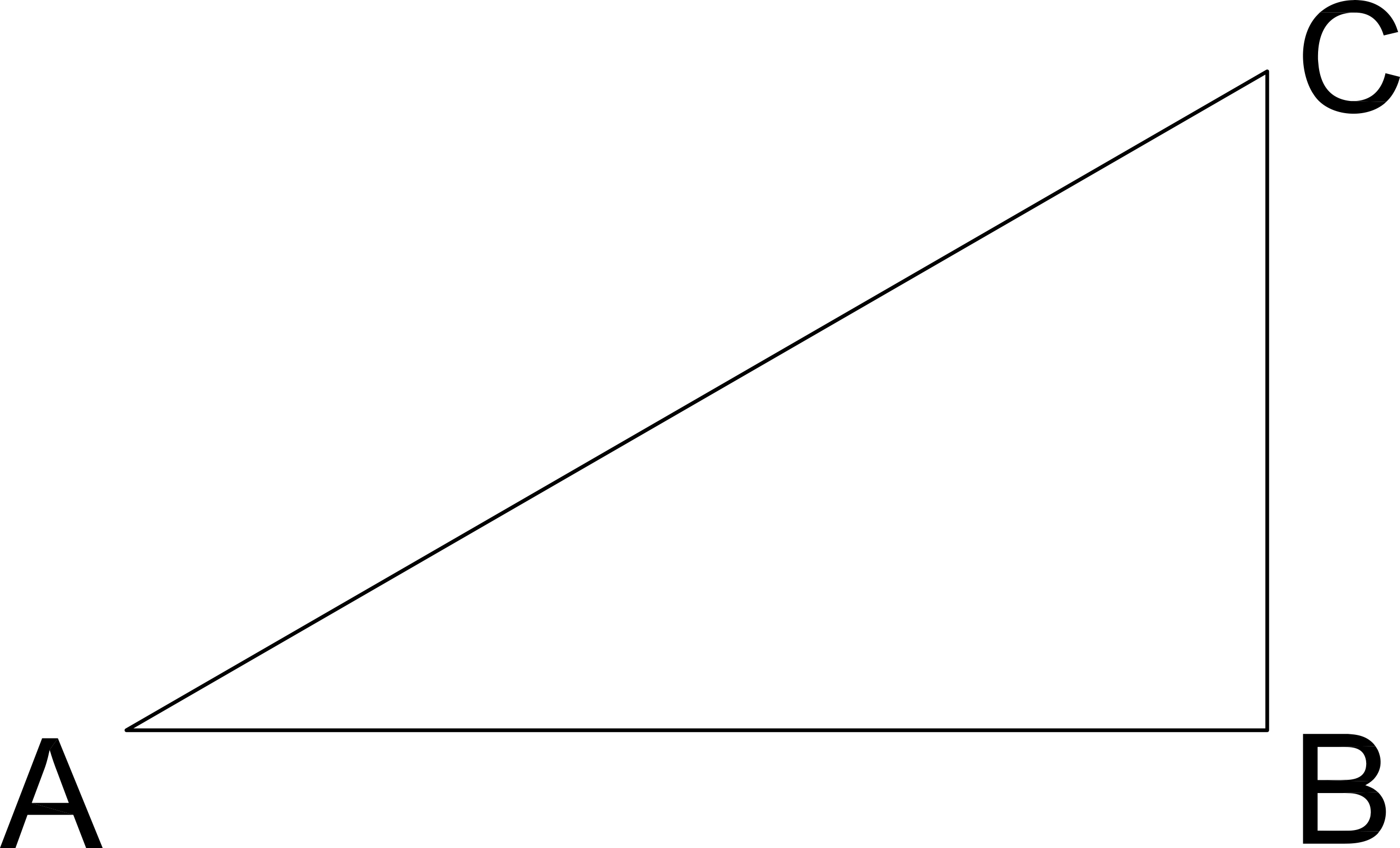If the length of CB is 6 and the angle C measures 45º, what is the length of AC in the given right triangle?

6√2

72

12√2

6

9

6√2

Explanation:

Pythagorean Theorum

AB2 + BC2 = AC2

If C is 45º then A is 45º, therefore AB = BC

AB2 + BC2 = AC2

62 + 62 = AC2

2*62 = AC2

AC = √(2*62) = 6√2

### Example Question #31 : How To Find The Length Of The Hypotenuse Of A Right Triangle : Pythagorean Theorem

You leave on a road trip driving due North from Savannah, Georgia, at 8am.  You drive for 5 hours at 60mph and then head due East for 2 hours at 50mph.  After those 7 hours, how far are you Northeast from Savannah as the crow flies (in miles)?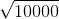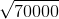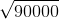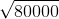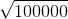Explanation:

Distance = hours * mph

North Distance = 5 hours * 60 mph = 300 miles

East Distance = 2 hours * 50 mph = 100 miles

Use Pythagorean Theorem to determine Northeast Distance

3002 + 1002 =NE2

90000  + 10000 = 100000 = NE2

NE = √100000

### Example Question #41 : How To Find The Length Of The Hypotenuse Of A Right Triangle : Pythagorean Theorem

A square garden has an area of 49 ft2. To the nearest foot, what is the diagonal distance across the garden?

10

7

11

9

8

10

Explanation:

Since the garden is square, the two sides are equal to the square root of the area, making each side 7 feet. Then, using the Pythagorean Theorem, set up the equation 7+ 7= the length of the diagonal squared. The length of the diagonal is the square root of 98, which is closest to 10.

### Example Question #42 : How To Find The Length Of The Hypotenuse Of A Right Triangle : Pythagorean Theorem

A man at the top of a lighthouse is watching birds through a telescope. He spots a pelican 5 miles due north of the lighthouse. The pelican flies due west for 12 miles before resting on a buoy. What is the distance, in miles, from the pelican's current resting spot to the lighthouse?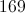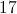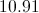Explanation:

We look at the 3 points of interest: the lighthouse, where the pelican started, and where the pelican ended. We can see that if we connect these 3 points with lines, they form a right triangle. (From due north, flying exactly west creates a 90 degree angle.) The three sides of the triangle are 5 miles, 13 miles and an unknown distance. Using the Pythagorean Theorem we get: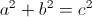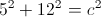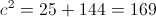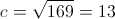### Example Question #81 : Sat Mathematics

An airplane is 8 miles west and 15 miles south of its destination.  Approximately how far is the plane from its destination, in miles?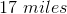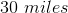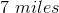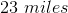Explanation:

A right triangle can be drawn between the airplane and its destination.

Destination

15 milesAirplane

8 miles

We can solve for the hypotenuse, x, of the triangle:

82 + 152 = x2

64 + 225 = x2

289 = x2

x = 17 miles

### Example Question #31 : How To Find The Length Of The Hypotenuse Of A Right Triangle : Pythagorean Theorem

An 8-foot-tall tree is perpendicular to the ground and casts a 6-foot shadow. What is the distance, to the nearest foot, from the top of the tree to the end of the shadow?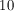Explanation:

In order to find the distance from the top of the tree to the end of the shadow, draw a right triangle with the height(tree) labeled as 8 and base(shadow) labeled as 6: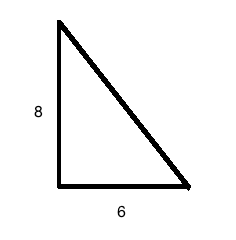From this diagram, you can see that the distance being asked for is the hypotenuse. From here, you can either use the Pythagorean Theorem: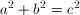or you can notice that this is simililar to a 3-4-5 triangle. Since the lengths are just increased by a factor of 2, the hypotenuse that is normally 5 would be 10.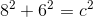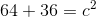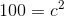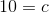### Example Question #81 : Geometry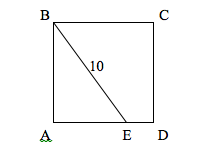In the figure above,is a square and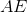is three times the length of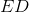. What is the area of?Explanation:

Assigning the length of ED the value of x, the value of AE will be 3x. That makes the entire side AD equal to 4x. Since the figure is a square, all four sides will be equal to 4x. Also, since the figure is a square, then angle A of triangle ABE is a right angle. That gives triangle ABE sides of 3x, 4x and 10. Using the Pythagorean theorem:

(3x)2 + (4x)2 = 102

9x2 + 16x2 = 100

25x2 = 100

x2 = 4

x = 2

With x = 2, each side of the square is 4x, or 8. The area of a square is length times width. In this case, that's 8 * 8, which is 64.

### All PSAT Math Resources